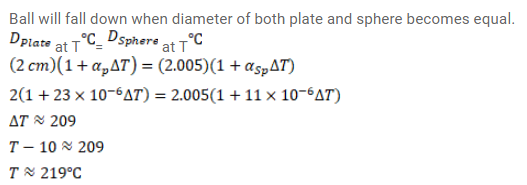# An aluminium plate fixed in a horizontal position has a hole diameterQuestion:

An aluminium plate fixed in a horizontal position has a hole diameter $2.000 \mathrm{~cm}$. A steel sphere of diameter $2.005 \mathrm{~cm}$ rests on this hole. All the lengths refer to a temperature of $10^{\circ} \mathrm{C} .$ The temperature of the entire system is slowly increased. At what temperature will the fall down? Coefficient of linear expansion of aluminium is $23^{\times 10^{-6}} /{ }^{\circ} \mathrm{C}$ and that of steel is $11^{\times 10^{-6}} /{ }^{\circ} \mathrm{C}$.

Solution: